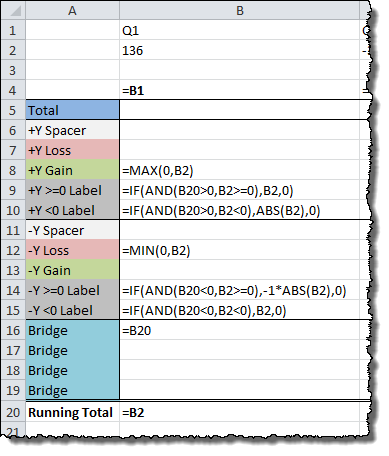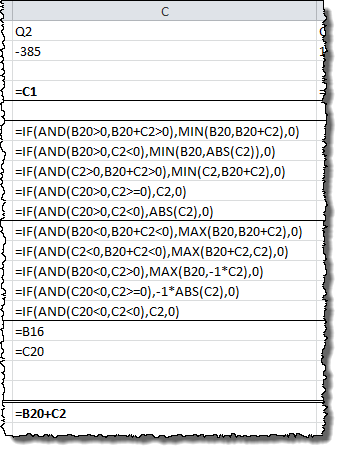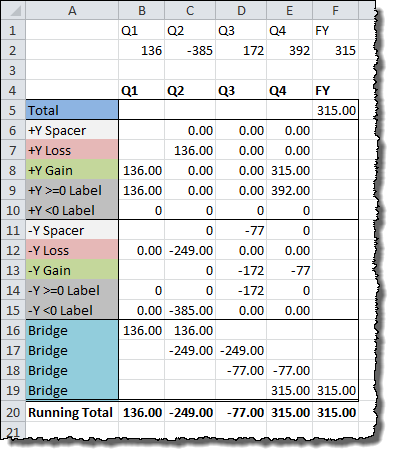# How to Create Waterfall Charts in Excel

## Filling in the Data Table

We’ll fill in the data table in two steps. The first column (Q1) will have one set of formulas, and then the rest will have another. These are the formulas in the first column:```=B1

=MAX(0,B2)
=IF(AND(B20>0,B2>=0),B2,0)
=IF(AND(B20>0,B2<0),ABS(B2),0)

=MIN(0,B2)

=IF(AND(B20<0,B2>=0),-1*ABS(B2),0)
=IF(AND(B20<0,B2<0),B2,0)
=B20

=B2```

The next column is as follows:```=C1

=IF(AND(B20>0,B20+C2>0),MIN(B20,B20+C2),0)
=IF(AND(B20>0,C2<0),MIN(B20,ABS(C2)),0) =IF(AND(C2>0,B20+C2>0),MIN(C2,B20+C2),0)
=IF(AND(C20>0,C2>=0),C2,0)
=IF(AND(C20>0,C2<0),ABS(C2),0)
=IF(AND(B20<0,B20+C2<0),MAX(B20,B20+C2),0)
=IF(AND(C2<0,B20+C2<0),MAX(B20+C2,C2),0)
=IF(AND(B20<0,C2>0),MAX(B20,-1*C2),0)
=IF(AND(C20<0,C2>=0),-1*ABS(C2),0)
=IF(AND(C20<0,C2<0),C2,0)
=B16
=C20

=B20+C2```

The columns after the first and second can be copied across. All that needs to be changed are the Bridge pair values. The final data table (using the example data) should look like this: